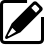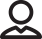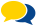## Spring Design

Design information for engineers, such as spring calculation formulas,

which are the basis of spring design, can be found here.

### Meaning of Symbols

The symbols used for spring design are shown in Table 1 below. The values of the longitudinal elastic modulus (E) are based on Table 2.

Table 1. Symbols and units used in calculations

Symbol Meaning of Symbol Unit
E Longitudinal elastic modulus N/mm2
b Material width mm
t Plate thickness mm
l Total length mm
M Moment N・mm
k Spring constant N・mm/rad
σ Stress N/mm2

Table 2.Longitudinal elastic modulus：E（N/m㎡）

Material E Value
Spring steel material 206×103
Stainless steel SUS302

SUS304

SUS631

186×103
186×103
196×103
Nickel-silver wire 108×103
Phosphor bronze wire 98×103
Beryllium copper wire 127×103

### Basic Calculation Formulas Used For Design

The basic formula for calculating the spring constant when winding is given by the following formula.Also, the stress when any moment occurs can be calculated with the following formula.Product informationOur ProposalsPopular questions